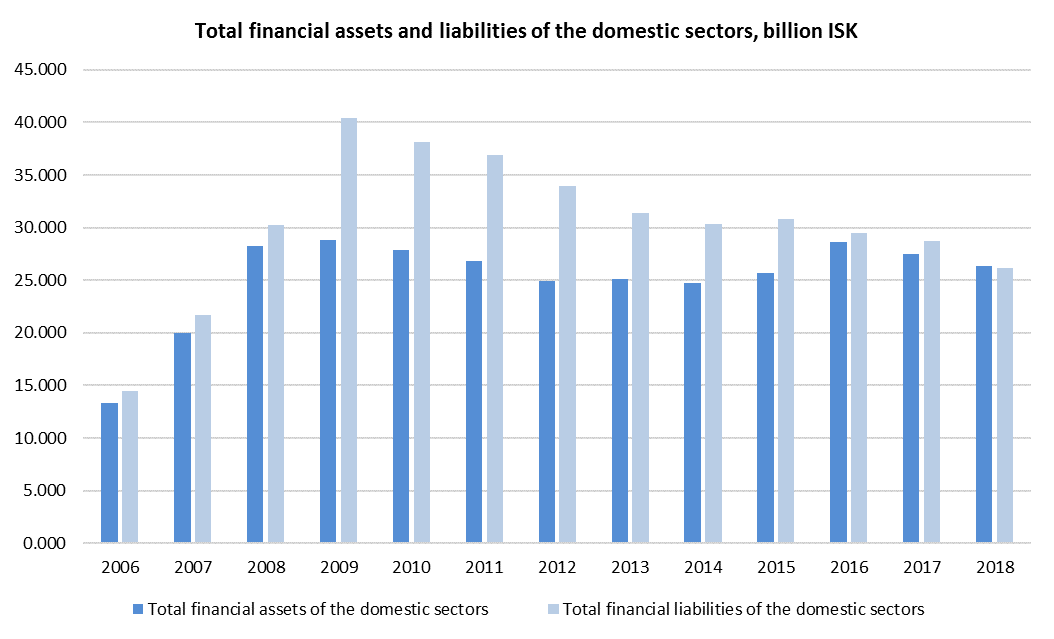Total financial liabilities of Iceland’s domestic sector stood at 26,116 billion ISK or 928% of Gross Domestic Product (GDP) in year-end 2018. Total financial assets stood at 26,253 billion ISK or 933% of GDP according to preliminary figures.Financial assets of households stood at 7,213 billion ISK, while financial liabilities stood at 2,167 billion ISK at the end of 2018, or 257% and 77% of GDP, respectively. Total financial assets increased by 4.4%, while liabilities increased by around 7.6% between 2017 and 2018.

Financial assets of non-financial corporations stood at 4,081 billion ISK according to initial estimates and decreased by 7.6% from the year 2017. However, financial liabilities stood at 7,736 billion ISK, increasing by 1% between years.

Total financial assets of financial corporations stood at 13,281 billion ISK, decreasing by 0.6% between years. However, financial liabilities were 14,215 billion ISK and lowered by 1.7% from the year before.

At the end of 2018, the financial assets of the general government stood at 1,586 billion ISK or 56.4% of GDP, while liabilities stood at 1,990 billion ISK or 71% of GDP.

The foreign sector’s financial assets stood at 3,596 billion ISK or 128% of GDP, while the liabilities stood at 3,723 billion ISK or 132% of GDP. Financial assets increased by 9.1% and liabilities by 9.3% between 2017 and 2018.

Statistics Iceland, in collaboration with the Central Bank of Iceland, is currently working on improving the methodology of financial accounts. An overview of the main changes can be found below. At this time we are only presenting the changes in the year 2017 according to the new methodology. A revision of the years prior to 2017, including an extension of the time-series all the way back to 1997, will be completed next year and published in September 2020.

Statistics